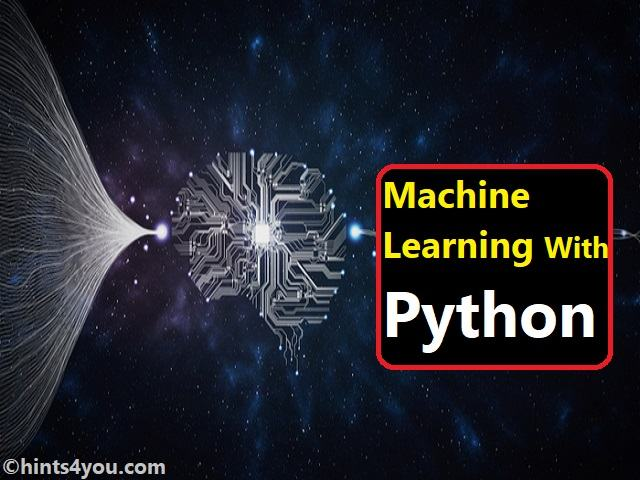# Basic Fundamentals Of Machine Learning With Python

## Machine Learning With Python:Machine Learning With Python

Today, We will get the information about machine learning with Python which is a really interesting topic. Machine Learning is an advanced process.

### Machine Learning is divided into three modules:

1.Supervised Learning It includes both dependent and independent variables. It is also called as Pattern Recognition. Example: Face and Character recognition. 2.Unsupervised Learning 3.Reinforcement Learning It has no output variable. It has only an input variable and has no target. It is based on a response. It is used in gaming applications. Example: Google search

### An algorithm in Supervised Learning:

1.Regression algorithm It is used to find the causal effect relationship. It is used to classify the records. 2.Classification algorithm
3.Decision Trees It uses Basket analysis. It is used to find the best solution to the problem in a diagrammatic way.

1.Clustering It is used for market segmentation.
2.Association Learning String Example: The recommendations we get in applications like Flip cart, Amazon uses machine learning.

### Machine Learning is used in various fields namely:

Bio Informatics Web mining Retail Telecommunications Medicine Finance Manufacturing

### Python Data Structures:

Dictionary: It is used for Indexing purposes declared in { }. It has the key: value pairs. Each pair is separated by a comma. Dictionary is mutable. It stores characters enclosed by “ ”. Tuple It is used to store multiple values, enclosed by ( ) and separated by a comma. Tuples are immutable which means the data can’t be updated once stored. List:
It has a maximum of 64*64 matrix limit in the array. The list is also used to store multiple values. The list is also mutable and enclosed by [ ]. Set: It is used to store a set of values without duplicates. The set is mutable and enclosed by { }.

### Packages in Python:

1) Numpy (Numerical Python) 2) Pandas It is used to store a large volume of data. It stores data in the form of data frames. 3) Matplotlib It is used for data visualization. It is a pictorial way of representation. 4) Scipy (Scientific Python) It is used for statistical analysis. 5) Sklearn (Scikit ) It is used in a machine learning algorithm.

### Why Python?

Open source so no license required. Example: defects per hour, number of children ii. Continuous Variable i.e undefined It has powerful libraries that can minimize the LOC. Variables:

It is of two types. a. Categorical Variable The values are predefined. Example: Eye color, marital status. b. Numerical Variable It represents quantities. It is further divided into 2 categories, It Discrete Variable i.e defined Example: weight, voltage

### The histogram is used to represent the frequency distribution of data.

Graphical Representation:
• Bar Graph is used when the x-axis is categorical and the y-axis is continuous.
• The line is used to finding the patterns and trends.
• The area is used to represent the distribution of the area.
• Pi is used to represent a 100% distribution of data. It is applicable only for categorical.
• Scatter Diagram is used when both x and y-axis is continuous. It is used to find the outliers.
Factor Analysis: A scree plot is a pictorial way of representing the factors. Factor analysis is an Unsupervised Learning Algorithm. The condition is all variables should be independent. The purpose of factor analysis is Dimension Reduction or Data Reduction or Data Reduction Technique. There are 2 eligibility tests in factor analysis namely KMO Test (Kaiser-Meyer-Olkin) and Barlett’s Test. If the KMO value is greater than 0.5 it is used to check whether the sample is adequate or not. In the Barlett test, the result should be 0.00. I suppose the KMO Test fails it means the sample is not sufficient that means, we need to collect more data. If the 2nd test fails, we need to identify the variable and remove it.
There are 2 methods namely Extraction Method and Rotation Method. The extract is used to extract the number of variables into a number of factors. In this, we use PCA Principal Component Analysis. Whichever variable greater than Eigenvalue is extracted as 1. The rotation method is used to remove the residual. In this, we use Varimax rotation.
Factor analysis has 2 important terms, factor loading-finding the correlation between the factor and the variable and factor matrix-factor values loaded in a tabular form.
In high/low graph x-axis is categorical and the y-axis is continuous in which each category in the x-axis represents min, max, and middle value. Boxplot is used to check whether the data is normally distributed or not. It also is used to find the outliers. Dual Axis is used when two y-axes sharing the common-axis. Cluster Analysis: It is Unsupervised learning. It is a grouping technique using Euclidean Distance. It is used for market segmentation. Methods used in this are Hierarchical –used to find the no of clusters and the no of clusters not predefined and Non-Hierarchical. Clusters (Also called K-Mean Clustering)- are used to understand the characteristics of each cluster and the number of clusters predefined, are represented using the Agglomeration schedule which is a tabular way of representation and Dendrogram which is a pictorial representation. The linkage methods are single linkage, complete linkage, and Average linkage. The mean of each cluster is called cluster centroid. Each and every respondent is a cluster membership. Regards, Hints 4 You. Thanks for giving me your precious time. We will meet again via the next post. 💬Contributed by: Maria Jovita Vaz Note: If you like Hints 4 You and would like to contribute, you can also write an article using hints4you.com/p/submit-guest-post or mail your article to hints4you.com@gmail.com. See your article appearing on the Hints4You main page and help other readers.
Please Improve this article if you find anything incorrect by the comments box which is available below post.